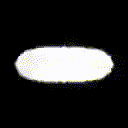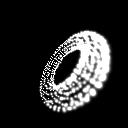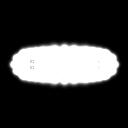# Affine transformation detection from images

Hello there. I’m wanted to know if what I’m doing seems sensible or correct? I’ve been using Tensorflow for some time and I’m looking at PyTorch as an alternative. In a nutshell, I have an image of a known solid object (a torus) represented as a point set. This is rotated around two axes (X and Z) and rendered to an image with a gaussian blur.

*I’d post the image here but this forum only allows one image per post (which seems a little low) *

The idea now, is given this set of points, can a neural net find the angles Rx and Rz that created this image?

My plan was initially to render an image, compare this generated image to the baseline and generate a loss that way. However, this didn’t work. I realised that one could transform a point from 3D to 2D pixel coordinates easily enough but then assigning this to an image and generating a loss function won’t work as you can’t get a derivative from an index-and-assign operation. Makes sense.

Instead, I found the function grid_sample and decided that I could generate pixel coordinates and check whether or not our generated pixel position has ‘hit’ a valid area (i.e is the sample white (1.o) or not).

I wrote some basic code to see how good this loss function is:

``````def test(points, rot_mat, mask) :

size = (128, 128) # N x C x H x W apparently
base = test_compare()
base = base.expand((1,1,size,size))
learning_rate = 0.1

ndc_mat = gen_ndc(size)
#rot_mat = gen_rot(x_rot, y_rot, z_rot)
trans_mat = gen_trans(0.0, 0.0, 2.0)
proj_mat = gen_perspective(math.radians(90), 1.0, 1.0, 10.0)
rot_mat.retain_grad() # Need this because it's not a leaf node?!?!

for i in range(200):

model_mat = torch.matmul(trans_mat, rot_mat)
o = torch.matmul(model_mat, points)
q = torch.matmul(proj_mat, o)
# Divide through by W seems to work with a handy mask and sum
w = torch.sum(w,1,keepdim=True)
r = q / w

s = r.narrow(1, 0, 2).reshape(1,1,-1,2)
output = F.grid_sample(base, s)
dtype=torch.float32)

criterion = torch.nn.MSELoss()
loss = criterion(output, gauss_point)
loss.backward(retain_graph=True)

#print("rot_mat", rot_mat)
#loss.backward(retain_graph=True)
splatted = splat(points, model_mat, proj_mat, ndc_mat, size)
img = Image.fromarray(np.uint8(splatted.detach().numpy() * 255))
img.save("torch" + str(i).zfill(3) + ".jpg", "JPEG")
return rot_mat

``````

For brevity I haven’t included the other functions (but maybe I’ll post the entire thing somewhere else).
The final result looks a little like this:Ultimately, this hasn’t really worked. One clue is the final rotation matrix looks like this:

``````tensor([[ 0.4880, -0.1858, -0.0320, -1.2093],
[ 0.1985,  1.2921, -0.0137, -0.1724],
[ 0.0349, -0.0112,  0.8019,  0.1691],
[ 0.0697, -0.0224, -0.3963,  1.3382]], grad_fn=<MmBackward>)

``````

Clearly that’s not correct as the last row should be 0,0,0,1 in the ideal case. I’ve clearly messed up with the maths somewhere. I realise this is quite a tricky problem and I’ve barely scratched the surface. I suppose my first question would be:

``````loss.backward(retain_graph=True)
``````

Why do I need retain_graph=True here? Without it, I get no .grad attached to my rot_mat. I thought that rot_mat would be a leaf node and would therefore have it’s gradients worked out and ready for me to apply?

Cheers
Ben

Original baseline image is here.Essentially, I’d like to get the rendered image as close to this one as possible

Sounds like an interesting project.
It seems you are trying to learn the rotation matrix, but apparently you get some invalid values in the last row.
Would it make sense to split the rotation and translation part of your transformation and try to learn the rotation then?
It wouldn’t be that performant, but maybe it’ll help the model to learn.

Hello there! Thanks for the reply!

I spoke with a colleague who suggested a much better solution for the point-to-image mapping. Firstly, I should say, I don’t know the correct terminology. I believe a 3D point is in the form [x, y, z, w] and a bitmap is of the form W x H x C where C is usually the colour depth / channels in this case, one channel. I thought I’d repeat it here because I’ve seen this question pop up a few times before (whilst I was looking for it) and I don’t think it’s been answered.

If one has a point and you want to see how it appears on the screen AND use this screen image as part of a loss function if needs to be differentiatable all the way through. To do this I’ve found the following:

1. Take your 3D point and pass it through your model -> view -> perspective -> W/divide -> ndc_to_screen matrices. This gives (Xs, Ys)
2. call torch.narrow() to remove z and w coordinates. This leaves you with a 2D screen coordinate
3. Create two matrices (2D tensors here) called XS and YS. These will be, I believe they are called, index matrices. They have the form (W,H). XS looks like:
[0,1,2,3… w]
[0,1,2,3…w]
.
.
[h]

You can call xs.permutate([1,0]) to get the ys matrix.

1. The clever bit. Expand your point (Xs, Ys) using expand_as(xs) and expand_as(ys) to give xe and ye.
2. Subtract xs from xe and the same for ys and ye. You now have two matrices that correspond to the offsets from the point you passed in. The smallest value will be 0 and that’s where your point lives in the final image.
3. use any function you like to combine these two matrices into a final image. One could invert, multiply and normalise for points, or use a gaussian function.

All of that is differentiable and can be used as as cost function against another image (least I hope so!)
I’ve improved the code which makes asking questions a bit easier.
The main problem is the chain of gradients and backward step. Ideally, I want to have just two variables - Xrot and Zrot. For some reason, I can only get the rot_mat to update and it’s very wrong. Not only that I have to retain the graph and retain the gradient on the rot_mat. None of this is ideal.

``````def new_gauss(ex, ey, xs, ys, sigma):
torch.exp(-((ex - xs)**2 + (ey-ys)**2)/(2*sigma**2)), dim=0),\
0.0,1.0)

size = (128, 128) # N x C x H x W apparently
base = test_compare()
learning_rate = 0.1
sigma = 2.5

# Create a square array of X positions
numbers = list(range(0,size))
square = [ numbers for x in numbers]
cube = []
for i in range(0,points.shape):
cube.append(square)
xs = torch.tensor(cube, dtype = torch.float32)
# Now move it around to get the equivalent Y positions
# These two matrices will be used with our Point coord
ys = xs.permute([0, 2, 1])
ndc_mat = gen_ndc(size)
rot_mat = gen_rot(x_rot, y_rot, z_rot)
trans_mat = gen_trans(0.0, 0.0, 2.0)
proj_mat = gen_perspective(math.radians(90), 1.0, 1.0, 10.0)

for i in range(100):
rot_mat.retain_grad() # Need this because it's not a leaf node?!?!
model_mat = torch.matmul(trans_mat, rot_mat)
o = torch.matmul(model_mat, points)
q = torch.matmul(proj_mat, o)
# Divide through by W seems to work with a handy mask and sum
w = torch.sum(w, 1, keepdim=True)
r = q / w
s = torch.matmul(ndc_mat, r)
t = s.narrow(1, 0, 2)

px = t.narrow(1, 0, 1)
py = t.narrow(1, 1, 1)

ex = px.expand(points.shape, xs.shape, xs.shape)
ey = py.expand(points.shape, ys.shape, ys.shape)

model = new_gauss(ex, ey, xs, ys, sigma)
save_image(model)
loss = ((base-model)**2).sum()
loss.backward(retain_graph=True)

splatted = splat(points, model_mat, proj_mat, ndc_mat, size)
img = Image.fromarray(np.uint8(splatted.detach().numpy() * 255))
img.save("torch" + str(i).zfill(3) + ".jpg", "JPEG")

return rot_mat

``````

The result of the intitial step is pretty good, which shows the conversion into a set of pytorch matrix functions is working. Not much to look at but it is indeed, a torus edge on.So the main question I have - if I’m getting the gradients to flow back, why do I need retain graph and is there a reason why parts of the rotation matrix that shouldn’t be messed with are being altered? Is there a way to only be concerned with X and Z rotation angles here?

Cheers
Ben

To solve the problem with `retain_graph`, can you try cloning and detaching `rot_mat` in the beginning of `for` loop? i.e., I feel that you have a long chain of `rot_map` in memory which makes it difficult to trace. Can you try this inside `for` loop?

``````for i in range(100):
rot_mat = rot_mat.detach().clone()
....
``````

Hey there! Thanks for the reply. Your code suggestion does indeed solve the retain graph problem. I’m still attempting to track back the gradients to the intiial x_rot and z_rot parameters.

PyTorch seems to have a lot of side-effects and things going on in the background. I’ll need to be careful. It would be nice to get a bit more explicit and hunt down exactly what is going on.

So I thought I’d simplify things a little and I think I’ve hit the problem. I suspect this is something to do with the dynamic graph nature of PyTorch. The following code comes up with a reasonable result:

``````import torch

use_cuda = True
device = torch.device("cuda" if use_cuda else "cpu")

x_rot = torch.tensor([0.0], dtype=torch.float32,\

x_sin = torch.sin(x_rot)
x_cos = torch.cos(x_rot)

[0,0,-1,0],
[0,1,0,0],
[0,0,0,0]], dtype=torch.float32, device=device)

[0,1,0,0],
[0,0,1,0],
[0,0,0,0]], dtype=torch.float32, device=device)

base = torch.tensor([[1,0,0,0],\
[0,0,0,0],\
[0,0,0,0],\
[0,0,0,1]], dtype=torch.float32, device=device)

print(rot_x)
learning_rate = 0.1

# Base is rotated by 90 around X
base = torch.tensor([[1,0,0,0],
[0,0,-1,0],
[0,1,0,0],
[0,0,0,1]], dtype=torch.float32, device=device)

loss = ((rot_x-base)**2).sum()
loss.backward()

print(x_rot)

``````

However, if I attempt to put a loop around the following lines:

``````
for i in range(20):
loss = ((rot_x-base)**2).sum()
loss.backward()

print(x_rot)

``````

We get the problem where no gradient appears on x_rot. I suspect I need to find out more about loops and the backing graph that pytorch creates.

So I think I’ve solved it. It’s a little verbose but this loss function appears to work for rotation matrices. I figure I’ll post this here in case it’s useful to folks?
Thanks for the help ya’all!

``````""" A short program to test the loss function for a rotation matrix.
We take in 3 angles and attempt to move one matrix to another.
We default to the GPU.
"""

import torch
import math

def gpu(myfunc):
def inner_func(*args, **kwargs):
device = torch.device("cuda")
tt = myfunc(*args, **kwargs)
tt.to(device)
return tt
return inner_func

learning_rate = 0.1
use_cuda = True
device = torch.device("cuda" if use_cuda else "cpu")

x_rot = torch.tensor([0.0], dtype=torch.float32,\

y_rot = torch.tensor([0.0], dtype=torch.float32,\

z_rot = torch.tensor([0.0], dtype=torch.float32,\

def make_rot(x_rot, y_rot, z_rot, device="cpu") :
""" Make a rotation matrix from 3 tensors of dimension 
representing the angle in radians around X, Y and Z axes in this
order. It seems very verbose but this really does seem to work.
"""
x_sin = torch.sin(x_rot)
x_cos = torch.cos(x_rot)

y_sin = torch.sin(y_rot)
y_cos = torch.cos(y_rot)

z_sin = torch.sin(z_rot)
z_cos = torch.cos(z_rot)

[0,0,-1,0],
[0,1,0,0],
[0,0,0,0]], dtype=torch.float32, device = device)

[0,1,0,0],
[0,0,1,0],
[0,0,0,0]], dtype=torch.float32, device = device)

[0,0,0,0],
[-1,0,0,0],
[0,0,0,0]], dtype=torch.float32, device = device)

[0,0,0,0],
[0,0,1,0],
[0,0,0,0]], dtype=torch.float32, device = device)

[1,0,0,0],
[0,0,0,0],
[0,0,0,0]], dtype=torch.float32, device = device)

[0,1,0,0],
[0,0,0,0],
[0,0,0,0]], dtype=torch.float32, device = device)

base_x = torch.tensor([[1,0,0,0],\
[0,0,0,0],\
[0,0,0,0],\
[0,0,0,1]], dtype=torch.float32, device = device)

base_y = torch.tensor([[0,0,0,0],\
[0,1,0,0],\
[0,0,0,0],\
[0,0,0,1]], dtype=torch.float32, device = device)

base_z = torch.tensor([[0,0,0,0],\
[0,0,0,0],\
[0,0,1,0],\
[0,0,0,1]], dtype=torch.float32, device = device)

# Why does this line screw up but two matmuls are fine? :S
#rot_mat = rot_x * rot_y * rot_z
tmat = torch.matmul(rot_x, rot_y)
rot_mat = torch.matmul(tmat, rot_z)
return rot_mat

if __name__ == "__main__":

base = make_rot(\
device = device)

print(base)

for i in range(50):
print("Step", i)
rot_mat = make_rot(x_rot, y_rot, z_rot, device)
print(rot_mat)
loss = ((rot_mat-base)**2).sum()
loss.backward()
print(loss)